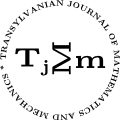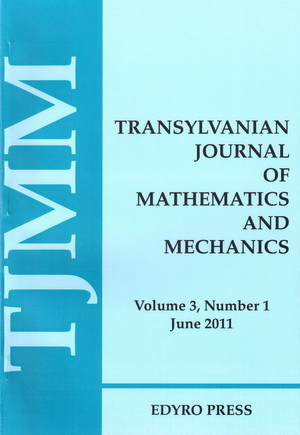ISSN: 2067-239X
ISSN(on-line): 2067-239X

Indexed in:
Mathematical Reviews
Zentralblatt MATH

Front cover# Volume 10 (2018), Number 2

## Existence of Nonoscillatory Solutions of Delay Dynamic Equations

### Author(s): ABDELOUAHEB ARDJOUNI and AHCENE DJOUDI

Abstract: This article concerns the existence of nonoscillatory solutions for a dynamic equation on time scales. The main tool employed here is the Schauder’s ﬁxed point theorem. The results obtained here extend the work of Kubjatkova, Olach and Stoberova .

## An Unique and Symple Mathematical Model for the Universe Expansion and the Introduction of Some Switching Coefficients for its Deﬁnition

### Author(s): MALVINA BAICA and MIRCEA CARDU

Abstract: In this paper the authors revisit the Mathematical Models of the Universe Expansion previously deﬁned in  and  and point out some of their shortcomings. In order to remove these shortcomings we elaborate a simple Mathematical Model which is valid for the entire domain of the no dimensional time.

## A New Subclass of Harmonic Univalent Functions Deﬁned By a Linear Operator

### Author(s): HASAN BAYRAM and SIBEL YALÇIN

Abstract: In the present paper, we investigate some basic properties of a subclass of harmonic functions deﬁned by multiplier transformations. Such as, coeﬃcient inequalities, distortion bounds, convolutions, convex combinations and extreme points.

## Erratum to "Inequalities of Ostrowski and Simpson Type for Mappings of Two Variables With Bounded Variation and Applications"

### Author(s): H. BUDAK and M.Z. SARIKAYA

Abstract: In recent publication , authors prove two inequalities of Ostrowski type and Simpson type for functions of two variables with bounded variation. However, there are some minor errors appeared in main results arising out of using a inexact lemma. These errors are corrected in this Erratum.

## Some Series of Distributionally Integrable Functions

Abstract: We study several properties of series of distributionally integrable functions. We consider the case of a nowhere dense closed subset $K$ of $\left[a,b\right],$ with $a,b\in K,$ and complementary open intervals $\left({a}_{n},{b}_{n}\right).$ We prove that if $f$ is distributionally integrable in $\left[a,b\right]$ and if the primitives of order $m$ of $f,$ ${F}_{m},$ are such that their restrictions ${{F}_{m}|}_{K}$ are absolutely continuous in $K$ for all $m\ge 1,$ then $f{\chi }_{K}$ is Lebesgue integrable and $f{\chi }_{\left[a,b\right]}=f{\chi }_{K}+\sum _{n=1}^{\infty }f{\chi }_{\left[{a}_{n},{b}_{n}\right]}\phantom{\rule{0.3em}{0ex}},$ in the space ${\mathsc{𝒟}}^{\prime }\left(ℝ\right).$ We also prove that under these conditions several related results hold, in particular the generalized integration by parts formula ${\int }_{K}f\left(x\right)\varphi \left(x\right)\phantom{\rule{0.3em}{0ex}}\mathrm{d}x=-{\int }_{K}F\left(x\right){\varphi }^{\prime }\left(x\right)\phantom{\rule{0.3em}{0ex}}\mathrm{d}x+{F\varphi |}_{a}^{b}-\sum _{n=1}^{\infty }{F\varphi |}_{{a}_{n}}^{{b}_{n}}\phantom{\rule{0.3em}{0ex}},$ where $\varphi$ is a test function. Furthermore, we prove that ${\int }_{a}^{b}f\left(x\right)\phantom{\rule{0.3em}{0ex}}\mathrm{d}x={\int }_{K}f\left(x\right)\phantom{\rule{0.3em}{0ex}}\mathrm{d}x+\sum _{n=1}^{\infty }{\int }_{{a}_{n}}^{{b}_{n}}f\left(x\right)\phantom{\rule{0.3em}{0ex}}\mathrm{d}x\phantom{\rule{0.3em}{0ex}},$ if $f$ is distributionally integrable and the restriction of its ﬁrst order primitive to $K$ is absolutely continuous. We give examples that show that the formulas may fail when the hypotheses are not satisﬁed.

## A New Proof of a Caristi Type Fixed Point Theorem

### Author(s): YUQIANG FENG and YUANYUAN WANG

Abstract: In this note, we give a new proof of a Caristi type fixed point theorem without using Zorn’s lemma and transfinite induction.

## Generalized Blasius Equation: Existence, Uniqueness and Analytical Approximation Solution

### Author(s): SAMY MZIOU, LAZHAR BOUGOFFA and RANDOLPH C. RACH

Abstract: In this paper, we consider the following nonlinear boundary value problem with varying coeﬃcients for the generalized Blasius equation proposed in  as an open problem: $\begin{array}{rcll}\left\{\begin{array}{ccc}\hfill {\left(a\left(\eta \right){f}^{″}\left(\eta \right)\right)}^{\prime }+f\left(\eta \right){f}^{″}\left(\eta \right)& \hfill =\hfill & 0,\phantom{\rule{1em}{0ex}}0<\eta <\infty ,\hfill \\ \hfill f\left(0\right)=0,\phantom{\rule{1em}{0ex}}{f}^{\prime }\left(0\right)=0,\phantom{\rule{1em}{0ex}}{f}^{\prime }\left(\infty \right)& \hfill =\hfill & 1,\hfill \end{array}\right\& & & \text{}\end{array}$ where $a\left(\eta \right)>0$ is a positive function of $\eta$. This problem contains the classical Blasius equation as a special case when $a\left(\eta \right)$ is a constant, that is, $a\left(\eta \right)=a$. Our first attempt is to discuss, using the classical fixed point theorem, the existence and uniqueness of the solution of this nonlinear problem. Some properties for $a\left(\eta \right)$ constant are also exhibited. Then, a highly accurate analytic approximation of the solution of this problem is provided by using the Adomian decomposition method.

## Analysis of the Evolution and Correlation Between Gross Net Salary and Consumer Price Index

### Author(s): ANAMARIA POPESCU

Abstract: This paper presents an econometric analysis of the ratio between the gross net salary and the consumer price. The work begins with a historical overview. The calculation statistics for the two variables will be presented and the relation between the variables will be further studied. Next we will ﬁnd the regression equation by applying the regression function in EXCEL, Regression. Also, the hypothesis of homoscedasticity will be veriﬁed by using the graphical method and applying the Durbin-Watson test. If the relationship is directly proportional (the increase of the variable 1 involves the increase of the variable 2, the decrease of the variable 1 involves the decrease of the variable 2), or inversely proportional (the increase of the variable 1 leads to the decrease of the variable 2). Finally, we will present the conclusions and references.

## A Three-Dimensional Generalized BVP of Thermoelasticity for a Layer: Green’s Functions and Integration Formula

### Author(s): ȘEREMET VICTOR

Abstract: This study devoted to constructing displacements Green’s functions for a generalized 3D BVP of uncoupled thermoelasticity within a layer by using harmonic integral representations method, proposed in the previous works. First are derived structural formulas for main thermoelastic Green’s functions for displacements, expressed via respective Green’s functions for Poisson’s equations. These structural formulas satisfy the equations of thermoelasticity with respect to point of application the heat source and the nonhomogeneous Poisson’s equation with respect to point of response in which the thermoelastic displacements appeared. In addition, they satisfy boundary conditions for temperature Green’s function with respect to point application the displacements and to mechanical boundary conditions with respect to point of application the heat source. The ﬁnal analytical expressions for displacements Green’s functions obtained on the base of mentioned above structural formulas for four 3D BVPs of thermoelasticity for the layer contain Bessel functions of the zero-order and the second type.

## Some New Hermite-Hadamard Type Inequalities for Functions Whose Derivatives Are Operator $\left(\alpha ,m\right)$-Preinvex

### Author(s): ERDAL ÜNLÜYOL and HÜMEYRA KARBUZ

Abstract: In this paper, it is introduced operator $\left(\alpha ,m\right)$-preinvex function in Hilbert spaces. And then it is obtained some new Hermite-Hadamard type inequalities for functions whose absolute value of derivatives are operator $\left(\alpha ,m\right)$-preinvex.

## Relations on Bi-Periodic Jacobsthal Sequence

### Author(s): S. UYGUN, H. KARATAS and E. AKINCI

Abstract: Following the new generalization of the Jacobsthal sequence defined by Uygun and Owusu  as ${\stackrel{̂}{ȷ}}_{n}=a{\stackrel{̂}{ȷ}}_{n-1}+2{\stackrel{̂}{ȷ}}_{n-2}$, when $n$ is even and ${\stackrel{̂}{ȷ}}_{n}=b{\stackrel{̂}{ȷ}}_{n-1}+2{\stackrel{̂}{ȷ}}_{n-2}$, when $n$ is odd, with initial conditions ${\stackrel{̂}{ȷ}}_{0}=0,{\stackrel{̂}{ȷ}}_{1}=a$ for all values of $n\ge 2$. In this paper, through a much broader study on this new generalization, particularly considering its Binet formula, some numerous new identities and properties of this sequence are investigated.